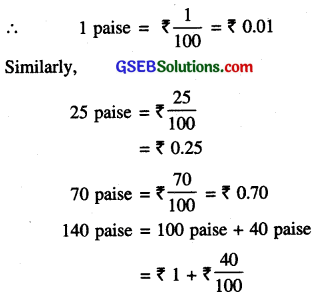# GSEB Class 6 Maths Notes Chapter 8 Decimals

This GSEB Class 6 Maths Notes Chapter 8 Decimals covers all the important topics and concepts as mentioned in the chapter.

## Decimals Class 6 GSEB Notes

Introduction:
In earlier classes we have learnt about decimals. The word decimal comes from Latin word ‘Decern’ meaning 10. In this class we shall study decimals as an extension of place value table as fractions and addition and subtraction of decimals.

Decimal Fractions:
The fractional numbers whose denominators are 10 or multiple of 10 are called decimal fractions. A decimal fraction has two parts, whole number part and decimal part. A dot is put between these two parts called the decimal point.

Example:
In 72.521, whole number part is 72 and decimal part is 521.
A decimal fraction can be written in expanded form as follows :
72.521 = 7 tens + 2 ones + 5 tenths + 2 hundredths + 1 thousandths
= 70 + 2 + $$\frac{5}{10}+\frac{2}{100}+\frac{1}{1000}$$

Tenths:
If an object is divided into 10 equal parts then each part is one-tenths of the whole.
It is written as $$\frac{1}{10}$$ or 0.1 and read as ‘zero point one.’Hundredths:
If an object is divided into 100 equal parts then each part is one-hundredths of the whole. It is written as $$\frac{1}{100}$$ or 0.01 and read as ‘zero point zero one. Thousandths. If an object is divided into 1000 equal parts then each part is one-thousandths of the whole. It is written as $$\frac{1}{1000}$$ or 0.001 and read as ‘zero point zero 1000 zero one.

In decimals, we have some distinctions which are as follows :

• A decimal number may contain a whole number and a decimal part .4, .23, 6.25 etc.
• If the decimal number consists only decimal part then zero can be written in the whole part
i. e. .3 = 0.3
.05 = 0.05 etc.
• If the decimal numbers consists only whole part then zero can be written in the decimal part.
i.e. 2 = 2.0
40 = 40.0 etc.

Conversion of Decimal Fraction into Fractional number

• Write the given number without the decimal point in the numerator.
• Write 1 in the denominator followed by as many Zeros as the decimal places.
• Write the resulting fraction in the lowest term.

Example.
3416 = $$\frac{3416}{100}=\frac{854}{25}$$

Conversion of Fractions to Decimal Numbers

• Count the number of Zeroes following 1 in the denominator.
• Count an equal number of places in the numerator starting from the unit digit, then place the decimal.

Example.
(a) $$\frac{732}{100}$$ = 7.32
(b) $$\frac{340}{1000}$$ = 0.340

Representation of Decimals on Number Line
Just like fractions, we can also represent the decimals on the number line.
Suppose we want to represent 0.4 on the number line. We know 0.4 is greater than 0 and less then 1 so it lies between 0 and 1.Also it has 4 tenths. Divide the distance between 0 and 1 into 10 equal parts. Then the 4th part represents 0.4. Similary other decimal fractions can be represented.

Like and Unlike Decimals
Like Decimals:
The decimals with the same number of decimal places are called like deci¬mals. e.g. 2.56,42.01,1.68,2.30 are like decimals, each having two places of decimals.

Unlike Decimals:
The decimals having different number of decimal places are called unlike decimals e.g. 2.1, 3.14,42.356 are un¬like decimal as they contain one, two, three decimal places respectively.Comparing Decimals
To compare decimals following steps are followed:

• Step 1. Convert unlike decimals to like decimals.
• Step 2. Compare the whole number part. Number with greater whole number part will be the greater decimal number.
• Step 3. If the whole number part is equal then compare the digits in the tenths place, the decimal num¬ber having greater number at the tenths place will be greater. If this is also same then check the hundredths place and so on.

Use of Decimals in Daily Life
Decimals are very useful in our daily life in form of money, weight, capacity etc. In this section, we shall learn about use of decimals in different fields of our life.

Money:
Conversion of paise into rupee.
As we know that
100 paise = ₹ 1= ₹ 1 + ₹ .40
= ₹ 1.40

Length‘s or Distance:
Conversion of cm into m.
We know that
10 cm = 1 m
1 cm = $$\frac{1}{100}$$ m
10cm = $$\frac{10}{100}$$m = 0.1 m
60cm = $$\frac{60}{100}$$ m = 060m
120cm = 100cm + 20cm
= 1m + 20cm
= 1m + $$\frac{20}{100}$$ m
= 1m + 20m
= 1.20 m.

Conversion of mm into cm.
We know that
10 mm = 1cm
1 mm = $$\frac{1}{10}$$cm = 0.1 cm

Conversion of metre into kilometre.
We know that
1 m = $$\frac{1}{1000}$$km = 0.001 km
Similarly, 56 m = $$\frac{56}{1000}$$m = 0.056 km

Weights:
Conversion of gram into kilogram.
We know that
1000 gm = 1 kg
∴ 1 gm= kg = 0.0001 kg
80gm = $$\frac{80}{1000}$$kg = 0.080 kg
1160gm = 1000gm + 160gm
= 1kg + $$\frac{160}{1000}$$kg
= 1kg + 0.160kg
= 1.160kg.

Capacity:
Conversion of millilitre into litre.
We know that
1000 ml = 1l
1 ml = $$\frac{1}{1000}$$ l
= 0.0011Addition of decimals numbers is same as addition of whole numbers. The only differ-ence is that we ensure that all decimal points will be in same column before addition. We use the following steps to add the decimals.

• Write the digits of the given decimal numbers one below the other such that all the decimal points are in the same vertical Line.